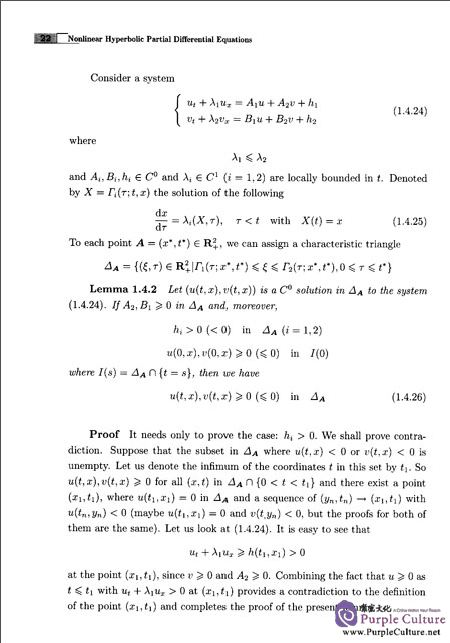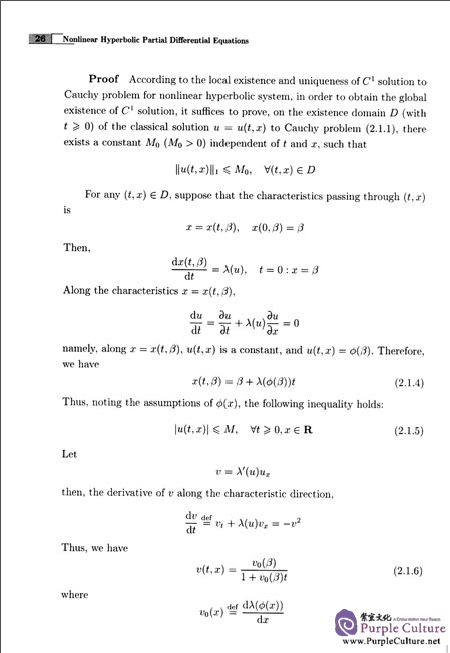FEEDBACK

#### Nonlinear Hyperbolic Partial Differential Equations

Price: \$10.33 \$7.26 (Save \$3.07)

Author: Wang Yuzhu; Liu Fagui;
Language: English
Format: 22.6 x 16.8 x 1.2 cm
Page: 210
Publication Date: 12/2016
ISBN: 9787302453765
Publisher: Tsinghua University Press
Preface
Chapter 1 Introduction
1.1 Intention and Significances
1.2 Basic Concepts
1.3 Some Examples
1.4 Preliminaries
Chapter 2 Cauchy Problem for Nonlinear Hyperbolic Systems in Diagonal Form
2.1 The Single Nonlinear Hyperbolic Equation
2.2 The Classical Solutions to Single Nonlinear Hyperbolic Equation
2.3 Nonlinear Hyperbolic Equations in Diagonal Form
Chapter 3 Singularities Caused by the Eigenvectors
3.1 Introduction
3.2 Completely Reducible Systems
3.32—Step Completely Reducible Systems
3.4 m（m＞2）—Step Completely Reducible Systems with Constant Eigenvalues
3.5 Non—completely Reducible Systems
3.6 Examples
Chapter 4 Hyperbolic Geometric Flow on Riemannian Surfaces
4.1 Introduction
4.2 Cauchy Problem for Hyperbolic Geometric Flow
4.3 Mixed Initial Boundary Value Problem for Hyperbolic Geometric Flow
4.4 Dissipative Hyperbolic Geometric Flow
4.5 Explicit Solutions
4.6 Radial Solutions to Hyperbolic Geometric Flow
Chapter 5 Life—Span of Classical Solutions to Hyperbolic Geometric Flow in Two Space Variables with Slow Decay Initial Data
5.1 Intention and Significances
5.2 Some Useful Lemmas
5.3 Lower Bound of Life—Span
Chapter 6 Nonlinear Hyperbolic Systems with Relaxation
6.1 Introduction
6.2 Global Classical Solutions
6.3 Applications
6.4 Convergence of Approximate Solutions
Chapter 7 Applications
7.1 One Dimensional Hydromagnetic Dynamics
7.2 Fluid Flow on a Pipe
7.3 Heat Conduction with Finite of Propagation
7.4 A Nonlinear Systems in Viscoelasticity
Bibliography
Index
##### Sample Pages Preview\$7.26